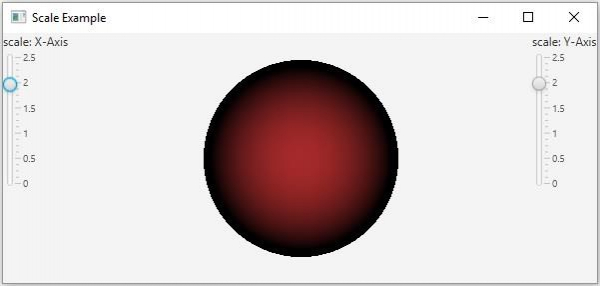# What is scale transformation in JavaFX?

A scale transform refers to minimizing or maximizing the size of an object. In JavaFX, you can scale a node using an object of the javafx.scene.transform.Translate class. Internally this class multiplies each unit in the coordinate system with the given factor.

This class contains six properties (double) type −

• Three (pivotZ, pivotY, pivotZ) specifying the x, y, z coordinates of the pivot point (about which the scaling occurs). You can set values to these properties using the setPivotX(), setPivotY() and, setPivotZ() methods respectively.

• Three properties specifying the scale factors along the x, y, and, z axes. You can set values to these properties using setX(), setY() and setZ() methods respectively.

Every node in JavaFX contains an observable list to hold all the transforms to be applied on a node. You can get this list using the getTransforms() method.

To scale a Node −

• Instantiate the Scale class.

• Set the scale factor and the pivot point using the respective setter methods.

• Get the list of transforms from the node (which you want to move) using the getTransforms() method.

• Add the above-created scale object to it.

• Add the node to the scene.

## Example

Following the JavaFX example demonstrates the scale transform. It contains a 2D geometric shape and two sliders, representing the x and y scale values. If you move the sliders the object scales along the chosen axis.

import javafx.application.Application;
import javafx.beans.value.ChangeListener;
import javafx.beans.value.ObservableValue;
import javafx.geometry.Orientation;
import javafx.scene.Scene;
import javafx.scene.control.Label;
import javafx.scene.control.Slider;
import javafx.scene.layout.BorderPane;
import javafx.scene.layout.VBox;
import javafx.scene.paint.Color;
import javafx.scene.paint.PhongMaterial;
import javafx.scene.shape.CullFace;
import javafx.scene.shape.DrawMode;
import javafx.scene.shape.Sphere;
import javafx.scene.transform.Scale;
import javafx.stage.Stage;
public class ScalingExample extends Application {
double x, y;
public void start(Stage stage) {
//Drawing a Sphere
Sphere sphere = new Sphere();
//Setting other properties
sphere.setCullFace(CullFace.BACK);
sphere.setDrawMode(DrawMode.FILL);
PhongMaterial material = new PhongMaterial();
material.setDiffuseColor(Color.BROWN);
sphere.setMaterial(material);
//Creating the scale transformation
Scale scale = new Scale();
//Setting the slider for the horizontal translation
Slider slider1 = new Slider(0, 2.5, 1);
slider1.setOrientation(Orientation.VERTICAL);
slider1.setShowTickLabels(true);
slider1.setShowTickMarks(true);
slider1.setMajorTickUnit(0.5);
slider1.setBlockIncrement(0.1);
public void changed(ObservableValue <?extends Number>observable, Number oldValue, Number newValue){
scale.setX((double) newValue);
}
});
//Setting the slider for the vertical translation
Slider slider2 = new Slider(0, 2.5, 1);
slider2.setOrientation(Orientation.VERTICAL);
slider2.setShowTickLabels(true);
slider2.setShowTickMarks(true);
slider2.setMajorTickUnit(0.5);
slider2.setBlockIncrement(0.1);
public void changed(ObservableValue <?extends Number>observable, Number oldValue, Number newValue){
scale.setY((double) newValue);
}
});
//Adding the scale transformation to the sphere
//Creating the pane
BorderPane pane = new BorderPane();
pane.setLeft(new VBox(new Label("scale: X-Axis"), slider1));
pane.setRight(new VBox(new Label("scale: Y-Axis"), slider2));
pane.setCenter(sphere);
//Preparing the scene
Scene scene = new Scene(pane, 595, 250);
stage.setTitle("Scale Example");
stage.setScene(scene);
stage.show();
}
public static void main(String args[]){
launch(args);
}
}

## Output